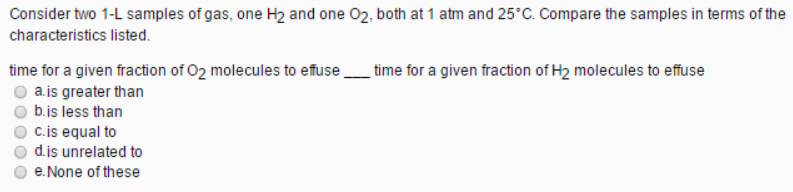# Problem: Consider two 1-L samples of gas, one H2 and one O2, both at 1 atm and 25°C. Compare the samples in terms of the characteristics listed. time for a given fraction of O2 molecules to effuse____time for a given fraction of H2 molecules to effuse a. is greater thanb. is less than c. is equal to d. is unrelated to e. None of these

###### FREE Expert Solution###### Problem Details

Consider two 1-L samples of gas, one H2 and one O2, both at 1 atm and 25°C. Compare the samples in terms of the characteristics listed.

time for a given fraction of O2 molecules to effuse____time for a given fraction of H2 molecules to effuse

a. is greater than

b. is less than

c. is equal to

d. is unrelated to

e. None of these